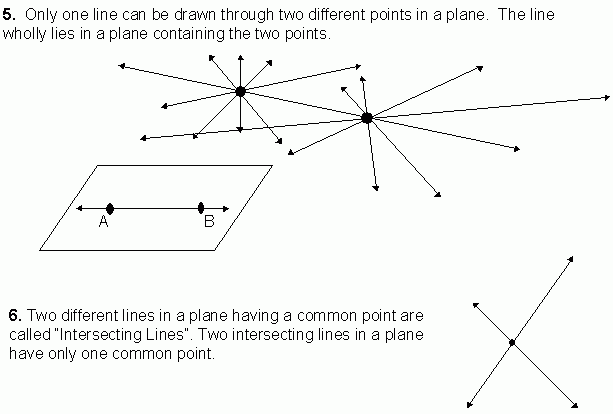Email us to get an instant 20% discount on highly effective K-12 Math & English kwizNET Programs!

#### Online Quiz (WorksheetABCD)

Questions Per Quiz = 2 4 6 8 10

### Geometry1.2 Basic Geometrical Concepts - Parallel Lines, Collinear Points and PlaneFor videos and additional resources on this topic, please see: MyndBook.com/go.php?to=207_885 Directions: Answer the following questions. Also on a sheet of paper draw a collinear points, non-collinear points and parallel and intersecting lines.
 Q 1: Number of lines that can be drawn through collinear points is _____.onetwoinfinity Q 2: If C is not a point on the line AB, then A, B, C are called _____ points.collinearlinearnon-collinear Q 3: A, B, C and D are four points such that not any three of them are on the same line. Then number of lines that can be drawn through them taking two points at a time is _____.twofoursix Q 4: Six points are on a paper such that not any three of them are collinear. Then number of lines that can be drawn joining two points at a time is _____.51015 Q 5: The surface of a box, the cover page of a book etc., are examples of parts of _____.linespointsplanes Q 6: A plane is a set of _______.pointslinesplanes Q 7: A plane is a flat surface extending _____ in all direction.finitelyindefinitelydefinitely Q 8: The flat surface of a white board gives an idea of a _____.planelinepoint Question 9: This question is available to subscribers only! Question 10: This question is available to subscribers only!